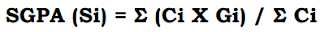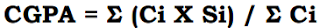### JNTUK Computation of CGPA and equivalent Percentage

JNTUK As per the B.Tech (R16) academic regulations of JNTUK, Kakinada, the following is the procedure for the computation of Cumulative Grade Point Average (CGPA).Where Ci is the number of credits of the ith course and Gi is the grade point scored by the student in the ith course.

The CGPA is calculated by taking into account all the courses undergone by a student in all the semesters of a programme, i.e.Where Si is the SGPA of the ith semester and Ci is the total number of credits in that semester.

The equivalent percentage can be calculated as per the below formula for the B.Tech students who obtains their degrees under R16 regulations ie. 2016-17 admitted batch onwards (2017-18 batch onwards for lateral entry scheme).

Equivalent Percentage = (CGPA – 0.75) X 10

Note: This Certificate can be downloaded from JNTUK examinations website www.jntukexams.net. No separate certificates will be issued to the students.

JNTUK Computation of CGPA and equivalent Percentage - Official Notification